The different stages of the amplifier require different voltage, absorb a different amount of current, and are more or less sensitive to noise (e.g. hum) produced by the power supply itself. The power supply unit has to consider these differences.

The power supply unit is composed of a power transformer, followed by a rectifier, and a sequence of smoothing filters dedicated to the different stages, as depicted in Figure 34.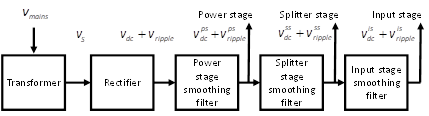Figure 34: Basic components of a Power Supply Unit.The power supply unit consists of chain of components containing a power supply transformer, a rectifier, and a sequence of smoothing filters.

The transformer takes as input, at its primary winding, the mains AC Vmains and returns, from its secondary winding, the AC voltage Vs to be given to the rectifier. The rectifier converts the received AC voltage into a DC voltage Vdc plus a residual AC voltage Vripple called the ripple voltage. This is due to the fact that there is no ideal rectifier, and a residual AC ripple voltage always remains on top of the wanted DC voltage. The sequence of smoothing filters, following the rectifier, have the purpose of both reducing the DC voltage, to the value needed by the corresponding stage, and reducing the ripple voltage, to a value tolerated by the stage itself.

In the following, we will first discuss rectifiers circuits, then we will discuss smoothing filters.

### 5.1.1    Rectifiers

Mains voltage has to be adapted to what needed by the vacuum tubes. For instance, mains voltage in Europe is 230V. This is generally not enough for most tubes, which often need more voltage. In addition, mains voltage is AC, while vacuum tubes require DC. Therefore, a step-up power transformer is first needed to bring the mains voltage up to the needed voltage. Then, the rectifier converts the AC current produced by the transformer into a DC current.

Figure 35 shows the schema of three very common types of transformers and rectifiers combinations. In the figure, RL represents the load of the power supply.

• The half wave rectifier schema, at the top of the figure, rectifies the AC voltage VS produced by the secondary of the transformer, using a single diode. The diode conducts only during the positive half cycles of VS. Therefore, the voltage waveform, produced by this rectifier, has the same shape than VS during the positive half cycles and it is zero during the negative half cycles.
• The full wave rectifier uses a centre-tapped transformer and two diodes. The centre tap is connected to ground. The two diodes have common cathodes, and anodes connected to the two ends of the transformer. The AC voltage VS, between the centre-tap and each end of the transformer, is half than the voltage measured between the two ends themselves. The phases of the voltage measured between the centre tap and one transformer end is the inverse of the phase from the centre tap and the other end. Therefore, when one diode sees a positive half cycle, the other sees a negative half cycle, and vice versa. The result is that the output waveform has positive pulses during all half cycles.
• The full wave bridge rectifier uses four diodes to convert all half cycles of the AC voltage VS into positive pulses. The output waveform is the same that that produced by the full wave rectifier.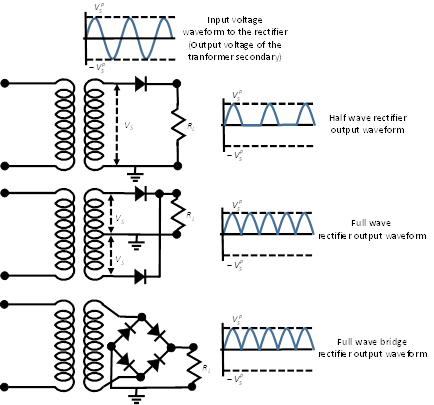Figure 35: Half wave, full wave, and full wave bridge rectifiers.The power transformer receives at its primary the mains AC voltage and produces, from its secondary, an AC output voltage VS. The rectifier eliminates negative pulses. RL represents the load of the power supply. The half wave rectifier, on top, just conducts during the positive half cycles. Therefore, the output voltage waveform has the same shape of VS, during the positive half cycles. It is 0 during the negative half cycles. The full wave rectifier, in the middle, uses a centre-tapped transformer. The AC output voltage VS is measured from the centre tap to the two ends. The output waveform has positive pulses during all half cycles. The full wave bridge rectifier does not need a centre-tapped transformer. It uses a bridge to convert the negative half cycles into positive. The resulting waveform is the same than that of the full wave rectifier.

All types of rectifier eliminate negative voltage coming from the VS waveform. However, the output voltage has a pulse waveform with a significant AC ripple component. The AC ripple voltage has a frequency equal to the mains frequency, for the half wave rectifier, and twice the mains frequency for the full wave rectifiers. The rectified voltage varies between zero and the peak voltage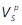. The peak voltage, reached by the pulses, is equal to the peak of the AC voltage Vs. If Vs is given as RMS voltage, then the peek voltage is.

The AC ripple voltage introduces in the output signal, produced by the amplifier, an unacceptable humming noise. A steadier DC voltage is needed and can be obtained by placing, after the rectifier, a reservoir capacitor and by using a sequence of smoothing filters, as discussed in next sections.

### 5.1.2    Reservoir capacitor

A very important component to complete a rectifier is the reservoir capacitor CR connected between the positive and the ground, as depicted in Figure 36. It significantly reduces the ripple voltage and returns a steadier DC voltage.

We explain the usage of the reservoir capacitor using a full wave rectifier. However, this discussion can also be generalized to other types of rectifiers.Figure 36: The reservoir capacitor.The reservoir capacitor CR connected between the positive and the ground significantly reduces the ripple of the rectified voltage.

The effect of the reservoir capacitor is shown in Figure 37. The blue waveform represents the rectifier output voltage, when no reservoir capacitor is used and no load is connected to the power supply. The red dotted waveform represents the positive pulse voltage of the two halves of the transformer secondary, with the reservoir capacitor and a load. The capacitor initially charges almost up to the peak voltage, as depicted by the red solid waveform. When the pulse voltage of the transformer secondary (red dotted waveform) decrease below the capacitor voltage, current no longer traverses the diode.  At this point, the capacitor feeds the load and slowly discharges. When the next pulse voltage is higher than the capacitor voltage, an intense current peak traverses the diode and the capacitor charges again quickly. The resulting voltage has a saw-tooth waveform. It goes quickly up during the charging phases. It goes slowly down during the discharging phases. The frequency is the same as the frequency of pulses arriving from the rectifier.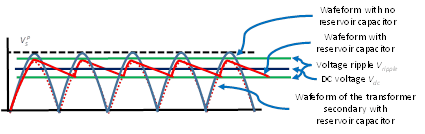Figure 37: Ripple voltage reduction with reservoir capacitor.The reservoir capacitor is charged almost up to the peak voltage during each half cycle. Its voltage is depicted by the solid red waveform. The voltage produced by the transformer secondary with a reservoir capacitor is represented by the red dotted waveform. When this voltage is below the reservoir capacitor voltage, the capacitor feeds the load and start discharging. When the rectified voltage is again high enough, it recharges the reservoir capacitor. The voltage of the reservoir capacitor has saw-tooth like waveform. It goes quickly up during the charging phases. It goes slowly down during the discharging phases.

The figure shows that the peak voltage reached by the capacitor, as depicted by the red solid waveform, is lower than the peak voltageof the rectifier without reservoir capacitor and load. This depends on the speed at which the capacitor charges, which in turns depends on

• the reservoir capacitance,
• the transformer impedance,

With the reservoir capacitor a ripple voltage still occurs, even if it is much smaller than that produced by the rectifier alone. The ripple voltage is due to the charging and discharging phases of the reservoir capacitor. On one hand, charging depends on the transformer output impedance and reservoir capacitance. Low output transformer impedance and low capacitance increase the peak voltage ripple and reduce reservoir capacitor charging time. On the other hand, reservoir capacitor discharging depends on the load impedance, the ripple frequency, and again the reservoir capacitance. Large load impedance, high ripple frequency, and large reservoir capacitance reduce the discharging voltage drop.

The output voltage is the sum of a DC voltage plus an AC (saw-tooth) ripple voltage Vdc+Vripple. Both can be estimated with sufficient accuracy, using results of a study carried out by Shade, discussed later.

However, in order to estimate DC output voltage and ripple voltage we first need to estimate the transformer output impedance and the load impedance, represented by the amplifier itself.

### 5.1.3    Transformer output impedance

The transformer output impedance Rs can be made explicit using an equivalent circuit where we place two resistors RS at the two transformer’s ends, as in Figure 38. Two components contribute to the resistance Rs. The first, Rsec-wind, is the secondary winding resistance. The second, Rprim-wind, is the primary winding resistance, reflected to the secondary. Since we are using a centre-tapped transformer, where contribution to form the output voltage is given by one transformer section at time, we need to consider as Rsec-wind just the resistance between the tap and one transformer end. The primary winding resistance, reflected to the secondary is equal to the primary winding resistance times the square of the ratio between the output voltage VS and input voltage Vmains (see Section 4.1.2 for discussion on the impedance reflected by transformers). RS is obtained as the sum of these two components:.

VS is the voltage between the centre tap and one transformer end.Figure 38: Transformer output resistance made explicit in an equivalent circuit.To make the transformer resistance explicit we draw an equivalent circuit where two resistors RS connect the two transformer’s ends to the two diodes. RS includes the secondary winding resistance and the primary winding resistance reflected to the secondary.
 Example 16: Power supply transformer output impedance Suppose for instance the primary winding resistance is 4 ohms, the secondary winding resistance is 20 ohm, the mains voltage is 230V, and the secondary transformer output voltage Vs is 325V. We have that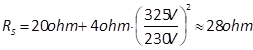.

The load RL is the resistance seen by the reservoir capacitor, which is the impedance offered to the power supply by all amplifier stages working in parallel. The impedance in each stage is the sum of the smoothing filter impedance, of that stage, plus the stage impedance itself. Provided the power stage is the first stage, the load RL can be roughly estimated, using the Ohm law, as the ratio between the voltage required by the power stage and the sum of the current absorbed by all stages. This approximation does not take into consideration the impedance of the power stage smoothing filter, which as discussed in Section 5.1.8, can only be computed once we know the DC output voltage of the rectifier. However, if the DC output voltage produced by the rectifier is not significantly higher than the voltage required by the power stage, the corresponding smoothing filter impedance is small, and the load seen by the reservoir does not significantly differ from this estimation.

 Example 17: Impedance offered by the amplifier to the power supply Suppose the power stage requires 400V and absorbs 80 mA, the phase splitter absorbs 1 mA, the input stage, also 1 mA. We have that. If we have a stereo amplifier, we have to divide this by 2, since the amplifier has double current absorption.

### 5.1.5    Estimation of the DC output voltage

The plot in Figure 39, taken by Shade’s work, puts in relationships all relevant variables and allows estimating the DC voltage Vdc for a full wave rectifier. Shade’s work reports the same graphs also for the other types of rectifiers. Every red plot corresponds to a percentage of the transformer impedance RS, with respect to the load resistance RL. Knowing the mains frequency fM, the reservoir capacitance CR, and the load RL, we fix 2πfMCRRL on the horizontal axis, and then we read the DC voltage Vdc as a percentage of the peak transformer voltage, on the corresponding red plot.

 Example 18: DC voltage output of a full wave rectifier Suppose for instance the load RL is 2.5K Ohm, the reservoir capacitor CR is 22 μF, RS is 28 ohms, the mains frequency fM is 50 Hz, and the secondary voltage is Vs=325V. The ratio RS/RL is 1.1% and 2πfMCRRL=17.2. Using the plot corresponding to a percentage of 1, in correspondence of 17.2 we have a percentage Vdc/around 92%. Since the peak voltage is, we have that the estimated DC output voltage is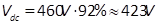.Figure 39: Determining the output DC voltage of a full wave rectifier.Relationships between output DC voltage, secondary transformer peak voltage, reservoir capacitance, mains frequency, load, and transformer impedance for a full wave rectifier. Every red curve corresponds to the percentage between RS and RL. The load RL is the impedance offered by the amplifier and the smoothing filters to the power supply. For each curve, knowing the mains frequency, the reservoir capacitance, and the load, we can obtain the ratio between the output DC voltage and the secondary transformer peak voltage.

### 5.1.6    Estimation of the ripple voltage

The plot in Figure 40, also taken by Shade’s work, allows us to estimate the output ripple voltage Vripple. As before, every plot corresponds to a different percentage ratio between the transformer impedance RS and the load resistance RL. Using the mains frequency fM, the reservoir capacitance CR, and the load RL, using one of the plots, we can obtain the ratio between the ripple voltage Vripple and the DC output voltage Vdc

 Example 19: Ripple voltage of a full wave rectifier Assuming the parameters obtained in Example 18, the plot corresponding to a ratio of 1, in correspondence of 17.2, gives us a percentage Vripple/Vdc around 4%. Therefore, the estimated ripple voltage is.

### 5.1.7    Estimation of the transformer secondary RMS current

Let us consider again, for this discussion, a full wave rectifier. Let IL be the current absorbed by the load. The current is fed, in turns, by the two sections of the transformer secondary through the two diodes. The average current Iavg flowing through each of the two transformer secondary sections and the two diodes is half the current traversing the load: Iavg = IL/2. However, previously we said that the transformer delivers current in intense peaks, during the charging phases of the reservoir capacitor. In fact, when the voltage of one of the transformer secondary sections is higher than the reservoir capacitor, the capacitor is quickly recharged with intense burst of current delivered by the transformer secondary through the two diodes, with a frequency twice the mains frequency. When the voltage of the transformer secondary section goes below the reservoir capacitor voltage, the diode does not conduce and the capacitor discharges, until the voltage of the other transformer secondary section is again higher than that of the reservoir capacitor, and so on. It is not easy to guess the RMS current IStraversing the two sections of the transformer secondary and the two diodes, given these current bursts.

Shade’s work also reports some guidelines for estimating the RMS current IS traversing the secondary winding of the power supply transformer and the rectifier diodes. The graph in Figure 41, derived by an equivalent graph from Shade’s work, puts in relationships all variable at hand, already used before. As before, every red plot corresponds to the ratio between the transformer impedance RS and the load resistance RL. Knowing the mains frequency fM, the reservoir capacitance CR, and the load RL, using one of the red plots, we can obtain the ratio between the RMS current IS and the current IL absorbed by the load.

 Example 20: RMS current in a transformer secondary of a full wave rectifier Let us use again the parameters obtained in Example 18. The plot corresponding to a ratio of 1, in correspondence of 17.2, gives us a percentage IS/IL around 1.5. In Example 18, the estimated output voltage was 423V. With a load of 2.5K Ohm the current is IL=423V/2.5K Ohm=170mA. The RMS current traversing the two transformer secondary sections and diodes is IS =1.5∙IL=1.5∙170mA=255mA.

The estimated value for IS can be used for determining the current rating of both the transformer and the diodes. Transformer and diodes should be chosen with a current rating higher than the estimated value, to guarantee safe operation also on extreme conditions. Generally, transformer and diode rating are chosen something like twice the obtained value. For instance, according to the value determined in Example 18 and Example 20, we can chose a centre tapped transformer rated for 650 V (325 V each section) at 500 mA (or simply 650V C.T. @ 500mA).

### 5.1.8    Smoothing filters

Smoothing filters are used to reduce DC voltage to that needed by each individual stage, and to further reduce the ripple voltage to a value tolerated by each stage. The initial stages tolerate much less ripple than the final stages. Reasonable values are:

• Input stage:                                      0.001%-0.002%
• Phase splitter stage:                     0.01%-0.05%
• Push-Pull power stage:                               0.5%-2%

A smoothing filter is in practice a low pass filter. It can be obtained using an inductor-capacitor network (LC low pass filter) or a resistor-capacitor network (RC low pass filter). Here we will discuss how a smoothing filter can be obtained using an RC network. What we discuss here can be easily extended to the case of an LC smoothing filter.

A simple schema for an RC smoothing filter is given in Figure 42. If this is the first smoothing filter, its input voltage arrives from the reservoir capacitor. Elsewhere, its input comes from the preceding smoothing filter. In both cases, the input voltage consists of a DC voltage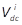plus an AC ripple voltage. The DC voltage is only affected by the resistor Rflt of the filter. The combined action of the resistor Rflt and the capacitor Cflt has effect on the AC ripple voltage.

Let us suppose that the stage of the amplifier powered by the filter (Stage 2 in Figure 42) requires a DC voltage V2 and absorbs a current I2. Let us also suppose that, the input DC voltage of the filter, or alternatively the DC voltage required by the previous amplifier stage (Stage 1 in Figure 42), is V1. Of course, V1 must be higher than V2. Finally, let us suppose that the next stages, powered by the power supply, absorb a current Inext.

The resistor Rflt has to produce a DC voltage drop of V– V2. The current that traverses the resistor is the current absorbed by the Stage 2 plus that absorbed by the next stages, which is I2 + Inext. To calculate the resistance of Rflt we can use the Ohm law: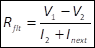.

 Example 21: Determining resistor for a power supply RC Smoothing filter Suppose, for instance, Stage 1 is the phase splitter stage, Stage 2 is the input stage, and no other stages are powered after the input stage. Suppose the voltage needed by the phase splitter is V1=380V, and the voltage needed by the input stage is V2=300V. Suppose the current in the input stage is 0.9mA, which is also the total current traversing the resistor, given that no other stages are powered next. In this case, we obtain that the resistor value must be Rflt=(380V-300V)/0.9mA=89K Ohm. The closest standard is 82K ohm and the dissipated power is P=(380V-300V) ∙0.9mA=0,072W.Figure 42: Power supply smoothing filters.A smoothing filter takes as input a DC voltage plus a ripple voltage and produces a reduced DC voltage with reduced ripple voltage as well. The input can be produced by the rectifier or by a proceeding smoothing filter. The output is used to give electric power to an amplifier stage. The input is generally also used to supply power to another stage of the amplifier.

Let us now consider the ripple voltage. The resistor Rflt and the capacitor Cflt form a voltage divider for AC voltage. Given that the capacitor is a reactive component we have that its impedance is,

where f is the frequency of the ripple voltage. Remember that, for a full wave rectifier, the ripple frequency f is twice the mains frequency.

Using the reactive voltage divider equation, we obtain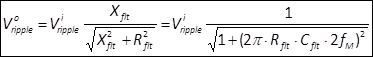.

 Example 22: Determining voltage ripple of a power supply RC Smoothing filter Suppose=90μV, Rflt=82K ohm and Cflt=22μF. In Europe, the mains frequency is 50 Hz, so the ripple frequency of a full wave rectifier is 100Hz. Therefore, we have that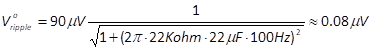.